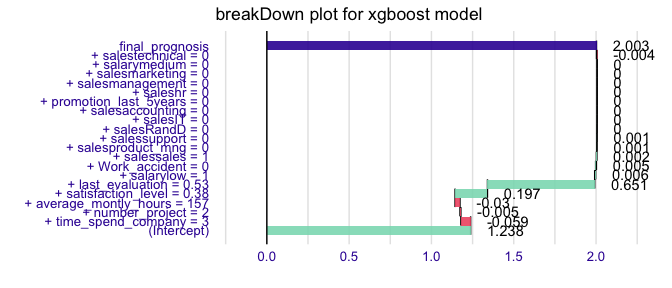How to use breakDown package for models created with xgboost

2020-04-05

This example demonstrates how to use the breakDown package for models created with the xgboost package.

library("breakDown")
library(xgboost)

model_martix_train <- model.matrix(left ~ . - 1, HR_data)
data_train <- xgb.DMatrix(model_martix_train, label = as.numeric(HR_data\$left))
param <- list(objective = "reg:linear")

HR_xgb_model <- xgb.train(param, data_train, nrounds = 50)
HR_xgb_model
#> ##### xgb.Booster
#> raw: 186.9 Kb
#> call:
#>   xgb.train(params = param, data = data_train, nrounds = 50)
#> params (as set within xgb.train):
#>   objective = "reg:linear", silent = "1"
#> xgb.attributes:
#>   niter
#> callbacks:
#>   cb.print.evaluation(period = print_every_n)
#> # of features: 19
#> niter: 50
#> nfeatures : 19

Now we are ready to call the broken() function.

library("breakDown")
nobs <- model_martix_train[1L, , drop = FALSE]

explain_2 <- broken(HR_xgb_model, new_observation = nobs,
data = model_martix_train)
explain_2
#>                              contribution
#> (Intercept)                         1.238
#> + time_spend_company = 3           -0.059
#> + number_project = 2               -0.005
#> + average_montly_hours = 157       -0.030
#> + satisfaction_level = 0.38         0.197
#> + last_evaluation = 0.53            0.651
#> + salarylow = 1                     0.006
#> + Work_accident = 0                 0.005
#> + salessales = 1                    0.002
#> + salesproduct_mng = 0              0.001
#> + salessupport = 0                  0.001
#> + salesRandD = 0                    0.000
#> + salesIT = 0                       0.000
#> + salesaccounting = 0               0.000
#> + promotion_last_5years = 0         0.000
#> + saleshr = 0                       0.000
#> + salesmanagement = 0               0.000
#> + salesmarketing = 0                0.000
#> + salarymedium = 0                  0.000
#> + salestechnical = 0               -0.004
#> final_prognosis                     2.003
#> baseline:  0

And plot it.

library(ggplot2)
plot(explain_2) + ggtitle("breakDown plot for xgboost model")